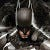Intersection Over Union

Source: Deep Learning on Medium

In the previous article, Indroduction to Object Detection, we have seen how a single object can be detected in an image by predicting a bounding box for the object. Like all Machine Learning Tasks, prediction of bounding box requires an accuracy metric to tell us how accurate the predictions are. In this article we will be discussing an accuracy metric that can be used for Object Detection.

I Owe You

Let us consider the following photo of a grumpy cat.

Now, in ideal case, an object detection algorithm should should be identifying him somewhat like this.

But, if an object detection algorithm outputs the following blue bounding box, how do we tell how much off the mark our prediction is???

In this case, we calculate IOU or Intersection Over Union for the following bounding boxes.

``IOU(Box1, Box2) = Intersection_Size(Box1, Box2) / Union_Size(Box1, Box2)``

Implementing IOU

The bounding box coordinates are in the form `(x, y, width, height)`. We will first calculate the width and height of the Intersection Box and size of Intersection will be area of the Intersection Box. We can get the Union size by subtracting the Intersection size from total area. All the code is part of the following Kaggle Notebook.

Code:

We will modify the visualization code to also show the respective IOU.